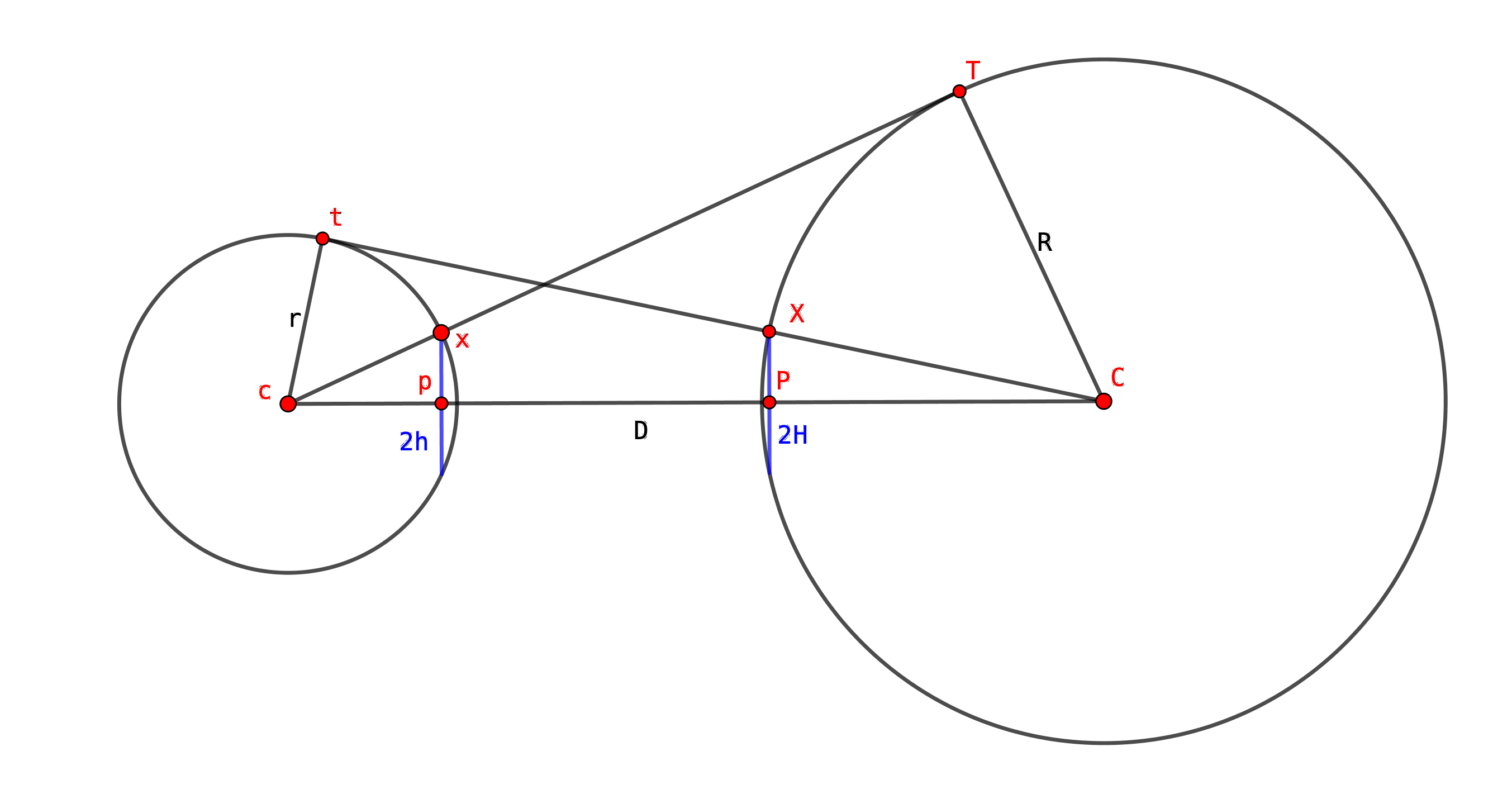A nice observation from Futility Closet:Draw two circles of any size and bracket them with tangents, as shown.

The chords in blue will always be equal.

I’m hardly going to let that pass by without a proof, now, am I? Spoilers below the line.

### My proof

• Definitions
• Let the distance between the centres be $D$.
• Let the radius of the left-hand circle be $r$ and that of the right-hand circle be $R$. Let their respective centres be $c$ and $C$.
• Let the length of the left-hand blue line be $2h$ and that of the right-hand blue line be $2H$. (I’ve put the 2 in because I’m going to halve them shortly.)
• Let the upper points of tangency be $t$ on the left circle and $T$ on the right circle.
• Let the upper points where the blue chords meet their circles be $x$ on the left and $X$ on the right.• Observations
• The left-hand blue chord crosses $cC$ at right angles; let the point where it does this be $p$.
• Similarly for the right-hand blue chord; let its crossing-point on $cC$ be $P$.
• Triangle $cxp$ is right-angled at $p$, and is similar to triangle $cCT$ - so $\frac{h}{r} = \frac{R}{D}$ .
• Similarly, $CXP$ is right-angled at $P$, and is similar to $CcT$ - so $\frac{H}{R} = \frac{r}{D}$ .
• Conclusion
• From , $h = \frac{rR}{D}$
• From , $H = \frac{rR}{D}$
• So $h=H$ and the two chords are equal.

* Edited 2020-08-17 to improve the diagram and change some colours. Thanks, Barney!